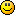## Discuss Scratch

### OS Window GUI holds on to each other.

Hmm.. I've seen this in "The Windows 10 Simulator" by colorgram. But I'm making an OS called HerbinOS, I cannot figure out how to get it to stick together like that. If anyone could help that'd be nice### OS Window GUI holds on to each other.

this might help

for the content and buttons inside the window:
`forevergo to x: ([x position v] of [Window v]) y: ([y position v] of [Window v])make sure to not center the exit and minimize buttons.end`

`(((((((((((((((((((((((((((hey I'm a reporter))))))))))))))))))))))`

### OS Window GUI holds on to each other.

also make sure that the window is draggable.

`(((((((((((((((((((((((((((hey I'm a reporter))))))))))))))))))))))`

Oh thanks!